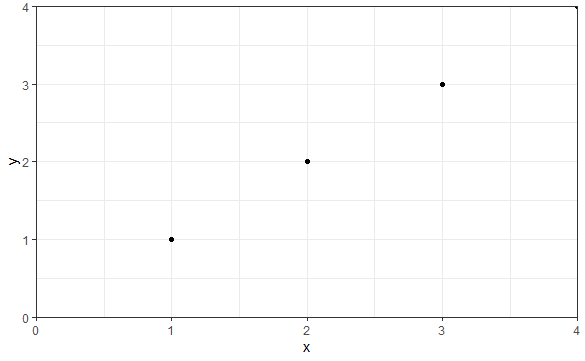# Force the origin to start at 0

1 view

How can I set the origin/interception of the y-axis and x-axis in ggplot2?

The line of the x-axis should be exactly at y=Z.

With Z=0 or another given value.

by (25.3k points)

To force the origin to start at zero, you can use the exapnd_limits function with scale_x_continuous, and scale_y_continuous as follows:

library("ggplot2")

df <- data.frame(x = 1:4, y = 1:4)

p <- ggplot(df, aes(x, y)) + geom_point()

p <- p + expand_limits(x = 0, y = 0) +theme_bw()

p + scale_x_continuous(expand = c(0, 0)) + scale_y_continuous(expand = c(0, 0))

Output: